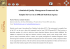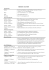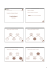# slides

## Transcription

slides
```Probabilistic Reasoning I
Chapter 14.1–3, Bayesian Networks
Chapter 14.1–3, Bayesian Networks
1
Warm-up: People are bad at probabilities
Suppose that you are worried that you might have a rare disease. You decide
to get tested, and suppose that the testing methods for this disease are
correct 99 percent of the time (in other words, if you have the disease, it
shows that you do with 99 percent probability, and if you don’t have the
disease, it shows that you do not with 99 percent probability). Suppose this
disease is actually quite rare, occurring randomly in the general population
in only one of every 10,000 people.
If your test results come back positive, what are your chances that you
actually have the disease?
Do you think it is approximately: (a) .99, (b) .90, (c) .10, or (d) .01?
Chapter 14.1–3, Bayesian Networks
2
Outline
♦ Syntax of Bayesian Networks
♦ Semantics of Bayesian Networks
♦ Efficient representation of conditional distributions
Chapter 14.1–3, Bayesian Networks
3
Example
Weather
Cavity
Toothache
Catch
W eather is independent of the other variables
T oothache and Catch are conditionally independent given Cavity
Topology of network encodes conditional independence assertions
Each node is annotated with probability information
The combination of the topology and the conditional distributions suffices
to specify the full joint distribution for all the variables
Chapter 14.1–3, Bayesian Networks
4
Bayesian networks
A Bayesian network is a directed graph in which each node
is annotated with quantitative probability information.
Syntax:
a set of nodes, one per random variable
a directed, acyclic graph (link ≈ “directly influences”)
a conditional distribution for each node given its parents: P(Xi|P arents(Xi))
that quantifies the effects of the parents on Xi
Example: I’m at work, neighbor John calls to say my alarm is ringing, but
neighbor Mary doesn’t call. Sometimes it’s set off by minor earthquakes. Is
there a burglar?
Given the evidence of who has or has not called, we would like to estimate
the probability of a burglary
Random variables:
Causal knowledge:
Chapter 14.1–3, Bayesian Networks
5
Example
I’m at work, neighbor John calls to say my alarm is ringing, but neighbor
Mary doesn’t call. Sometimes it’s set off by minor earthquakes. Is there a
burglar?
Variables: Burglar, Earthquake, Alarm, JohnCalls, M aryCalls
Network topology reflects “causal” knowledge:
– A burglar can set the alarm off
– An earthquake can set the alarm off
– The alarm can cause Mary to call
– The alarm can cause John to call
Laziness and Ignorance: MaryListeningMusic, JohnTelefonRings, ConfusingJohn
Chapter 14.1–3, Bayesian Networks
6
Conditional probability table
P(E)
P(B)
Burglary
B
E
P(A|B,E)
T
T
F
F
T
F
T
F
.95
.94
.29
.001
JohnCalls
Earthquake
.001
.002
Alarm
A
P(J|A)
T
F
.90
.05
A P(M|A)
MaryCalls
T
F
.70
.01
Sumarising uncertainty: i.e., the value 0.9 includes also the uncertainty for
JohnTelefonRings and ConfusingJohn
How many probabilities do we need?
Chapter 14.1–3, Bayesian Networks
7
14.2 Semantics of Bayesian Networks
What does a Bayesian network mean?
representation of the joint probability distribution (global semantics)
collection of conditional independence statements (local semantics)
Global semantics defines the full joint distribution
as the product of the local conditional distributions:
B
n
P (x1, . . . , xn) = Πi = 1P (xi|parents(Xi))
E
A
J
M
Recall: generic entry in the joint distribution is the probability of a conjunction of particular assignments to each variable, P (X1 = x1 ∧ ... ∧ Xn = xn).
e.g., P (j ∧ m ∧ a ∧ ¬b ∧ ¬e)
=
Chapter 14.1–3, Bayesian Networks
8
Global semantics
“Global” semantics defines the full joint distribution
as the product of the local conditional distributions:
B
n
P (x1, . . . , xn) = Πi = 1P (xi|parents(Xi))
e.g., P (j ∧ m ∧ a ∧ ¬b ∧ ¬e)
E
A
J
M
= P (j|a)P (m|a)P (a|¬b, ¬e)P (¬b)P (¬e)
= 0.9 × 0.7 × 0.001 × 0.999 × 0.998
≈ 0.00063
Chapter 14.1–3, Bayesian Networks
9
Constructing Bayesian networks
Need a method such that a series of locally testable assertions of
conditional independence guarantees the required global semantics
1. Choose an ordering of variables X1, . . . , Xn
2. For i = 1 to n
select parents from X1, . . . , Xi−1 such that
P(Xi|P arents(Xi)) = P(Xi|X1, . . . , Xi−1)
This choice of parents guarantees the global semantics (equivalence between
full joint distribution and bayesian networks):
n
P(X1, . . . , Xn) = Πi = 1P(Xi|X1, . . . , Xi−1) (chain rule)
n
= Πi = 1P(Xi|P arents(Xi)) (by construction)
Chapter 14.1–3, Bayesian Networks
10
Example
Suppose we choose the ordering M , J, A, B, E
MaryCalls
JohnCalls
P (J|M ) = P (J)?
Chapter 14.1–3, Bayesian Networks
11
Example
Suppose we choose the ordering M , J, A, B, E
MaryCalls
JohnCalls
Alarm
P (J|M ) = P (J)? No
P (A|J, M ) = P (A|J)? P (A|J, M ) = P (A)?
Chapter 14.1–3, Bayesian Networks
12
Example
Suppose we choose the ordering M , J, A, B, E
MaryCalls
JohnCalls
Alarm
Burglary
P (J|M ) = P (J)? No
P (A|J, M ) = P (A|J)? P (A|J, M ) = P (A)? No
P (B|A, J, M ) = P (B|A)?
P (B|A, J, M ) = P (B)?
Chapter 14.1–3, Bayesian Networks
13
Example
Suppose we choose the ordering M , J, A, B, E
MaryCalls
JohnCalls
Alarm
Burglary
Earthquake
P (J|M ) = P (J)? No
P (A|J, M ) = P (A|J)? P (A|J, M ) = P (A)? No
P (B|A, J, M ) = P (B|A)? Yes
P (B|A, J, M ) = P (B)? No
P (E|B, A, J, M ) = P (E|A)?
P (E|B, A, J, M ) = P (E|A, B)?
Chapter 14.1–3, Bayesian Networks
14
Example
Suppose we choose the ordering M , J, A, B, E
MaryCalls
JohnCalls
Alarm
Burglary
Earthquake
P (J|M ) = P (J)? No
P (A|J, M ) = P (A|J)? P (A|J, M ) = P (A)? No
P (B|A, J, M ) = P (B|A)? Yes
P (B|A, J, M ) = P (B)? No
P (E|B, A, J, M ) = P (E|A)? No
P (E|B, A, J, M ) = P (E|A, B)? Yes
Chapter 14.1–3, Bayesian Networks
15
Compactness and node ordering
MaryCalls
JohnCalls
Alarm
Burglary
Earthquake
Assessing conditional probabilities is hard in noncausal directions
(i.e, P (Earthquake|Burglary, Alarm)
Any order will work, but the resulting network will be more compact if the
variables are ordered such that causes precede effects
Network is less compact:
For burglary net:
For full joint distribution:
Chapter 14.1–3, Bayesian Networks
16
Compactness
Network is less compact: 1 + 2 + 4 + 2 + 4 = 13 numbers needed
For burglary net, 1 + 1 + 4 + 2 + 2 = 10 numbers
For full joint distribution 25 − 1 = 31
What about the node ordering: M,J,E,B,A? How many probabilities are
required?
A Conditional Probability Table (CPT) for Boolean Xi with k
Boolean parents has 2k rows for the combinations of parent values
B
E
A
Each row requires one number p for Xi = true
(the number for Xi = f alse is just 1 − p)
J
If each variable has no more than k parents,
the complete network requires O(n · 2k ) numbers
I.e., grows linearly with n, vs. O(2n) for the full joint distribution (k << n)
Chapter 14.1–3, Bayesian Networks
17
M
Local semantics
Local semantics: each node is conditionally independent
of its non-descendants given its parents
U1
Um
...
X
Z 1j
Z nj
Y1
...
Yn
JohnCalls is indepedent from:
Chapter 14.1–3, Bayesian Networks
18
Markov blanket
Each node is conditionally independent of all others given its
Markov blanket: parents + children + children’s parents
U1
Um
...
X
Z 1j
Z nj
Y1
...
Yn
Bulgary is indepedent from:
Chapter 14.1–3, Bayesian Networks
19
Example: Car diagnosis
Initial evidence: car won’t start
Testable variables (green), “broken, so fix it” variables (orange)
Hidden variables (gray) ensure sparse structure, reduce parameters
battery age
battery
battery
meter
lights
fanbelt
broken
alternator
broken
no charging
battery
flat
oil light
no oil
gas gauge
no gas
car won’t
start
fuel line
blocked
starter
broken
dipstick
Chapter 14.1–3, Bayesian Networks
20
Example: Car insurance
SocioEcon
Age
GoodStudent
ExtraCar
Mileage
RiskAversion
VehicleYear
SeniorTrain
MakeModel
DrivingSkill
DrivingHist
Antilock
DrivQuality
Airbag
Ruggedness
CarValue HomeBase
AntiTheft
Accident
Theft
OwnDamage
Cushioning
MedicalCost
OtherCost
LiabilityCost
OwnCost
PropertyCost
Chapter 14.1–3, Bayesian Networks
21
14.3 Efficient representation of cond. distribution
CPT grows exponentially with number of parents
CPT becomes infinite with continuous-valued parent or child
Deterministic nodes are the simplest case: its value is specified by the value
of parent
X = f (P arents(X)) for some function f
E.g., logical relationships
N orthAmerican ⇔ Canadian ∨ U S ∨ M exican
E.g., numerical relationships among continuous variables
∂Level
= inflow + precipitation - outflow - evaporation
∂t
Chapter 14.1–3, Bayesian Networks
22
Efficient representation of cond. distribution
Noisy-OR distributions model multiple noninteracting causes
Example: F ever ⇔ Cold ∨ F lu ∨ M alaria
Allows for uncertainty about the ability of each parent to cause the child to
be true (the causal relationship between parent and child may be inhibited)
Example: a patient could have a Cold, but not exhibit a Fever
Assumptions:
1) Parents U1 . . . Uk include all causes (can add leak node)
2) Independent failure probability qi for each cause alone
j
⇒ P (X|U1 . . . Uj , ¬Uj+1 . . . ¬Uk ) = 1 − Πi = 1qi
Chapter 14.1–3, Bayesian Networks
23
Efficient representation of cond. distribution
Fever is false if and only if all its parents are inhibited. The probability of
this is the product of the inhibition probabilities q for each parent
qcold = P (¬f ever|cold, ¬f lu, ¬malaria) = 0.6
qf lu = P (¬f ever|¬cold, f lu, ¬malaria) = 0.2
qmalaria = P (¬f ever|¬cold, ¬f lu, malaria) = 0.1
Cold
F
F
F
F
T
T
T
T
F lu
F
F
T
T
F
F
T
T
M alaria
F
T
F
T
F
T
F
T
P (F ever)
0.0
0.9
0.8
0.98
0.4
0.94
0.88
P (¬F ever)
1.0
0.1
0.2
0.02 = 0.2 × 0.1
0.6
0.06 = 0.6 × 0.1
0.12 = 0.6 × 0.2
Number of parameters linear in number of parents
Chapter 14.1–3, Bayesian Networks
24
Hybrid (discrete+continuous) networks
Discrete (Subsidy? and Buys?); continuous (Harvest and Cost)
Subsidy?
Harvest
Cost
Option 1: discretization—possibly large errors, large CPTs
Option 2: finitely parameterized canonical families
Option 3: define conditional distribution with a collection of instances
1) Continuous variable, discrete+continuous parents (e.g., Cost)
2) Discrete variable, continuous parents (e.g., Buys?)
Chapter 14.1–3, Bayesian Networks
25
Continuous child variables
Need one conditional density function for child variable given continuous
parents, for each possible assignment to discrete parents
Most common is the linear Gaussian model, e.g.,:
P (Cost = c|Harvest = h, Subsidy? = true)
= N (ath + bt, σt)(c)

2 


1
1  c − (ath + bt)  


√
=
exp − 
 

2
σt
σt 2π
Cost decreases as supply increases
Chapter 14.1–3, Bayesian Networks
26
Discrete variable with continuous parents
Probability of Buys? given Cost should be a “soft” threshold:
1
0.8
0.6
0.4
0.2
0
0
2
4
6
Cost c
8
10
12
Probit distribution uses integral of Gaussian:
Rx
N (0, 1)(x)dx
Φ(x) = −∞
P (Buys? = true | Cost = c) = Φ((−c + µ)/σ)
Chapter 14.1–3, Bayesian Networks
27
Why the probit?
1. It’s sort of the right shape
2. Can view as hard threshold whose location is subject to noise
Cost
Cost
Noise
Chapter 14.1–3, Bayesian Networks
28
Discrete variable contd.
Sigmoid (or logit) distribution also used in neural networks:
P (Buys? = true | Cost = c) =
1
1 + exp(−2 −c+µ
σ )
Sigmoid has similar shape to probit but much longer tails:
1
0.9
0.8
0.7
0.6
0.5
0.4
0.3
0.2
0.1
0
0
2
4
6
Cost c
8
10
12
Chapter 14.1–3, Bayesian Networks
29
Summary
A Bayesian network is a directed acyclic graph whose nodes correspond to
random variables; each node has a conditional distribution for the node,
given its parents
Bayesian networks provide a concise way to represent conditional independence relationships in the domain
A Bayesian network specifies a full joint distribution; each joint entry is
defined as the product of the corresponding entries in the local conditional
distributions. A Bayesian network is often exponentially smaller than an
explicitly enumerated joint distribution.
Hybrid Bayesian networks, which include both discrete and continuous variables, use a variety of canonical distributions.
Chapter 14.1–3, Bayesian Networks
30
Detecting pregnancy based on three tests
The first is a scanning test which has a false positive of 1% and a false
negative of 10%.
The second is a blood test, which detects progesterone with a false positive
of 10a false negative of 30%.
The third test is a urine test, which also detects progesterone with a false
positive of 10% and a false negative of 20%.
The probability of a detectable progesterone level is 90% given pregnancy,
and 1% given no pregnancy.
The probability of pregnancy after two positive tests is 0.364%.
Construct the Bayesian network and specify the conditional probability tables.
Chapter 14.1–3, Bayesian Networks
31
```

### Guide to Benefits Analysis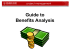### DON BOSCO INSTITUTE OF TECHNOLOGY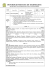### Document 6590118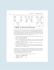### From CATS to SAT: Modeling Empirical Hardness to Understand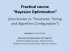### Bayesian Networks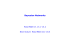### ë³´ê¸°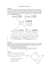### Spring 2015 UMKC Math & Stats Colloquium Series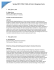### A Statistical Quality Management Framework for